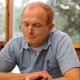# Purdy Cup round 3

Please see attached the results for tuesday and pairings for next week.

Also, Hobsons Bay Chess Club has been approached by the Chess Academy (which runs Junior coaching in the Wyndham and Point Cook areas) to participate in a simultaneous exhibition at the Featherbrook Shopping Centre during the Easter Long Weekend. This involves playing several members of the public, and will be used to promote the activities of both Chess Academy and Hobsons Bay Chess Club.

Regards

Steve

Round 3 results

``` 1 = Hogg,Dean          (2.0)-Nowak,Ruben          (2.0)= 1- 9= 1-0
2 = Paul,Nikash        (2.0)-Paul,Cijo            (2.0)=30- 4= 0-1
3 = Whitford,Matthew   (2.0)-McIntyre,Julian      (2.0)= 6-11= 0-1
4 = Saric,Sergey       (2.0)-Annapureddy,Rheyansh (1.5)=10- 8= 0-1
5 = Enano,Ronald       (1.5)-Matijas,Dejan        (1.0)= 5-12= 0-1
6 = Hogan,Steven       (1.0)-Katyal,Rohit         (1.0)= 7-26= 1-0
7 = De Rooy,Samuel     (1.0)-Galia,Aaryan         (1.0)=21-13= 1-0
8 = Maure,Eric         (1.0)-Cajes,Conrado        (1.0)=14-22= 0-1
9 = Kostakakis,Angelo  (1.0)-Krishnan,Anirudh     (1.0)=18-27= 0-1
10 = Davenport,John     (1.0)-Szuveges,Narelle S   (0.5)=34- 3= 0-1
11 = De Rooy,Jack       (1.0)-Wilkinson,Greg       (1.0)=20-33= 1-0
12 = Goh,Nicholas       (0.5)-Condick,Peter        (0.5)=24-15= 0-1
13 = Liu,Tina           (0.0)-Mahajan,Laksh        (0.0)=28-29= 0-1
14 = Kirk,Ian           (0.0)-Katyal,Krishiv       (0.0)=35-25= 1-0
15 = Cox,Aiden          (0.0)-Singh,Chanpreet      (0.0)=23-32= 0F-0F
16 = Davis,Tony J       (0.5)-( not paired )       (0.0)= 2- 0= 0
17 = Koci,Adem          (0.0)-( not paired )       (0.0)=16- 0= 0
18 = Clerk,Felix        (0.5)-( not paired )       (0.0)=17- 0= 0
19 = Clerk,Max          (1.0)-( not paired )       (0.0)=19- 0= 0
20 = Santoso,Crystin    (0.5)-( not paired )       (0.0)=31- 0= 0```